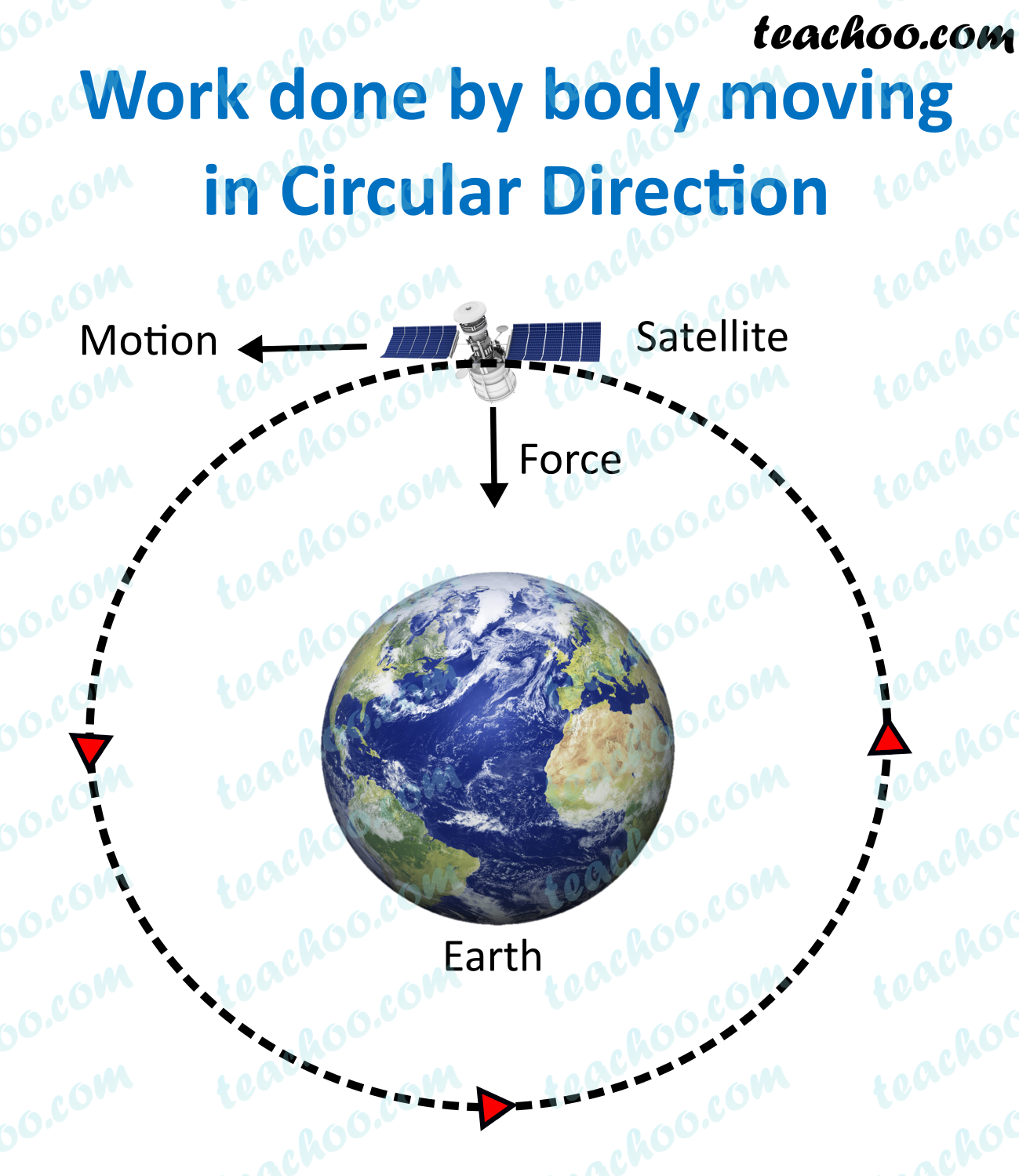NCERT Questions

Class 9
Chapter 11 Class 9 - Work and Energy (Term 2)

## What is the work done by the force of gravity on a satellite moving round the earth? Justify your answer.

###(adsbygoogle = window.adsbygoogle || []).push({});

Work is done when

1. Force is applied
2. Displacement takes place
3. The angle between the force and displacement is not 90 ° .

In case of the earth and a satellite,

Force experienced is gravitational force, which is towards the center along the radius

And displacement is along the tangent.

Since tangent is always perpendicular to the radius

Therefore, angle between force and the displacement is 90 degrees.

Hence, no work is done